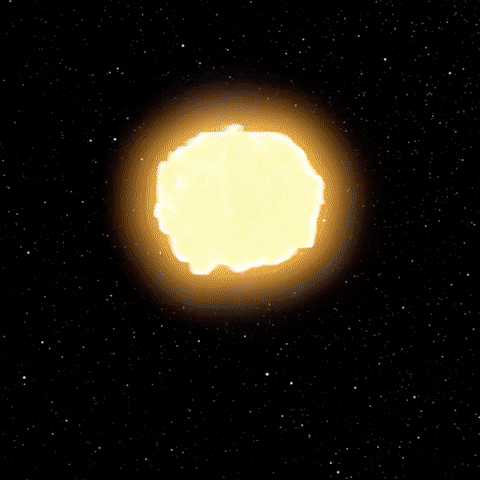# Separate vocals from a track using python

What I've planned to do in the past was learn how to separate vocals from a track programmatically and not depend on software-as-a-service to perform the separation of vocals from a track. This article shows how to separate the vocals of a song from the instruments using my new favorite library, Librosa. You can check out the Google Colab Notebook here.

The idea sparked when I wanted to separate individual tracks of a song, so I went to Product Hunt and discovered melody ml. This discovery started the urge to learn ML for music, hence the discovery of the Python library, librosa.

By the way, I ran out of RAM, which made my notebook explode.### Install and import dependencies

``````pip install librosa matplotlib IPython

import librosa
from librosa import display
import numpy as np
import IPython.display as ipd
import matplotlib as plt
``````

### Load and display the song.

I used My Last Serenade by KSE as I wondered how the growling or shouting parts of the song would come out.

``````y, sr = librosa.load('My Last Serenade.wav')
ipd.Audio(data=y[90*sr:110*sr], rate=sr)
``````

We slice a 20 second snippet in the chorus of the song. We show the audio using `ipd.Audio`(tbh, this is a bit exhausting). Photo is shown below because I couldn't find a way to upload audio here on DEV.### We separate a complex-valued spectrogram D into its magnitude (S) and phase (P) components, convert the time stamps into frames, plot the data, then display the full spectrogram of the data

``````S_full, phase = librosa.magphase(librosa.stft(y))
idx = slice(*librosa.time_to_frames([90*110], sr=sr))
fig, ax = plt.pyplot.subplots()
img = display.specshow(librosa.amplitude_to_db(S_full[:, idx], ref=np.max), y_axis='log', x_axis='time', sr=sr, ax=ax)
fig.colorbar(img, ax=ax)
``````

#### Line by line explanation

`S_full, phase = librosa.magphase(librosa.stft(y))` - we separate the magnitude and phase of the track using short-time fourier transform by representing a signal in the time-frequency domain by computing discrete Fourier Transforms(DFT)(y)

`idx = slice(*librosa.time_to_frames([90*110], sr=sr))` - slice a the part of the song then convert it to stft frames using the time_to_frames function of librosa

`img = display.specshow(librosa.amplitude_to_db(S_full[:, idx], ref=np.max), y_axis='log', x_axis='time', sr=sr, ax=ax)` - display the spectrogram of the 20 second sliced part of the song by converting the amplitude spectrogram to a dB-scaled spectrogram of the magnitude, then compares the magnitude and phase of the track and returns a new array containing the element-wise maxima then it plots the y and x axis

Below is the image of the spectrum:### Decomposing the spectrogram

``````S_filter = librosa.decompose.nn_filter(S_full, aggregate=np.median, metric='cosine', width=int(librosa.time_to_frames(2, sr=sr)))
S_filter = np.minimum(S_full, S_filter)
``````

#### Line by line explanation

`S_filter = librosa.decompose.nn_filter(S_full, aggregate=np.median, metric='cosine', width=int(librosa.time_to_frames(2, sr=sr)))` - we filter the vocals by its nearest neighbors, aggregate their median values, compare their frames using cosine similarity and contain those frames to be separated by 2 seconds and suppress other sounds from the spectrum

`S_filter = np.minimum(S_full, S_filter)` - we get the calculated data in the memory of the `S_full` and `S_filter` variables to get the minimum value.

### Display the background and foreground spectrum of the audio

``````margin_i, margin_v = 3, 11
power = 3

``````

#### Line by line explanation

`margin_i, margin_v = 3, 11` - we use margins to reduce loss in sound in the vocals and instrumented masks

`power = 3` - returns the soft mask computed in a numerically stable way

`S_foreground = mask_v * S_full` and `S_background = mask_i * S_full` - multiply the masks with the input spectrum to separate the components

### Plotting the full spectrum, background and foreground spectrum

``````fig, ax = plt.pyplot.subplots(nrows=3, sharex=True, sharey=True)
img = display.specshow(librosa.amplitude_to_db(S_full[:, idx], ref=np.max), y_axis='log', x_axis='time', sr=sr, ax=ax)
ax.set(title='Full Spectrum')
ax.label_outer()

display.specshow(librosa.amplitude_to_db(S_background[:, idx], ref=np.max), y_axis='log', x_axis='time', sr=sr, ax=ax)
ax.set(title='Background Spectrum')
ax.label_outer()

display.specshow(librosa.amplitude_to_db(S_foreground[:, idx], ref=np.max), y_axis='log', x_axis='time', sr=sr, ax=ax)
ax.set(title='Foreground Spectrum')
ax.label_outer()

fig.colorbar(img, ax=ax)
``````### Recover the foreground audio from the masked spectrogram and playback the audio

``````y_foreground = librosa.istft(S_foreground * phase)
ipd.Audio(data=y_foreground[90*sr:110*sr], rate=sr)
``````

#### Line by line explanation

`y_foreground = librosa.istft(S_foreground * phase)` - inverses the short-time fourier transform
`ipd.Audio(data=y_foreground[90*sr:110*sr], rate=sr)` - plays back the vocals from the track## Conclusion

This seemed easy at first thought and when I was reading the documentation but digging under the code made me realize that this idea was a little more complex. But, what made me continue was when I read about nearest neighbors in one part of the documentation which made me realize that I will be getting my hands on Machine Learning in the future with this library.